SARIMA-Based Medium- and Long-Term Load Forecasting

Chunlin Yin1, Kaihua Liu2, Qiangjian Zhang2, Kai Hu3, Zheng Yang1, Li Yang1 and Na Zhao1, 2, 4,*

1Electric Power Research Institute of Yunnan Power Grid, Kunming 650217, China
2Key Laboratory in Software Engineering of Yunnan Province, School of Software, Yunnan University, Kunming 650091, China
3Yunnan Power Grid Co., Ltd, Kunming 650011, China
4The Key Laboratory for Crop Production and Smart Agriculture of Yunnan Province, Kunming 650201, China
E-mail: zhaonayx@126.com
*Corresponding Author

Received 05 July 2022; Accepted 05 August 2022; Publication 31 January 2023

## Abstract

The operation and planning of power systems depend heavily on M-LTLF, which is complicated and nonlinear, making it challenging for conventional medium- and long-term forecasting models to produce reliable results. The SARIMA model is chosen for M-LTLF in this study, and the model’s parameters are tuned. This study takes the electricity consumption data of the whole Yunnan as the research object. Among them, the electricity consumption data from 2008 to 2018 is used as a training sample for fitting and analysis, and the electricity consumption of the whole province is predicted from 2019 to 2020. The end results demonstrate the viability and efficacy of the SARIMA model for M-LTLF.

Keywords: SARIMA, medium- and long-term load forecasting, time series models.

## 1 Introduction

Accurate power load forecasting is crucial to the functioning of the power systems because it forms the foundation for planning power layout and generation over the medium and long term. Even more so under China’s new economic normal, the new industrial system causes medium- and long-term loads to present complex asymmetric oscillating and variable characteristics, which significantly increases the difficulty of accurate power demand-side load forecasting . Medium- and long-term load forecasting (M-LTLF) requires a smaller amount of pertinent data and experiences a longer period of time than short-term load forecasting.

The study of medium- and long-term load demand forecasting is currently being pursued by a large number of academics. The fundamental concept is to build a mathematical model by identifying the time-series development pattern of the overall societal demand for energy and its historical correlation with other connected aspects, and to then realize the forecast through extrapolation. The following two aspects are included in the research on M-LTLF.

The Akaike information criterion (AIC) coefficient matrix, for instance, can be used to examine the ideal parameters and forecast data for the following two years based on 10 years of data. We have developed a method for medium and long-term power load forecasting using the SARIMA model. In this study, we thoroughly analyzed the entire set of data on electricity consumption in Yunnan Province. We discovered that the data had a good trend and strong seasonality, so after achieving reasonably good results with the ARIMA model, we decided to use the SARIMA model to capture its seasonal characteristics. To give a further verification of the effectiveness of the method, we used three models, ARIMA, HOLT_WINTER and LSTM to forecast the whole electricity consumption data of Yunnan Province with the SARIMA model used in this paper and made a side-by-side comparison in terms of accuracy, and finally proved that the method we put up is effective in medium- and long-term electricity load forecasting.

## 2 Theories and Methods

### 2.1 ARIMA Model

Box and Jenkins  proposed the ARIMA model in the 1970s, which can describe and predict time series very well. The entire load of each cluster was then predicted using it, and the load prediction error was examined. This is how the ARIMA (p, d, q) model is expressed:

 $yt$ $=λ1⁢yt-1+λ2⁢yt-2+⋯+λp⁢yt-p+ξt$ $-μ1⁢ξt-1-μ2⁢ξt-2-⋯-μp⁢ξt-p$ (1)

Where $ξt$ is the white noise, $λ$ and $μ$ are the coefficients.

The ARIMA model has two parts.

(1) Autoregressive model part

 $yt=λ1⁢yt-1+λ2⁢yt-2+⋯+λp⁢yt-p+ξt$ (2)

(2) Moving average model part

 $yt=ξt-μ1⁢ξt-1-μ2⁢ξt-2-⋯-μp⁢ξt-p$ (3)

The two parts of the ARIMA model reflect the characteristics of the power system at the past moment and the impact of disturbance on the model state at the current moment. If the time series is not smooth, a differential pre-processing is necessary since the ARIMA model performs better when predicting stationary time series data. The differencing order is d.

The optimal ARIMA model is constructed for $Si,t(i=1,2,…,K)$, and the future load values of them are predicted separately and summed to obtain the final power system load forecast.

Step 1 The pre-processed series $Si,t$ is first tested to see if it is smooth or not, and then the non-stable series is converted into a smooth series using difference computation.

Step 2 The next step is to construct an ARIMA(p,d,q) product model for each stationary time series data. Limited by the sequence length, here p and q are restricted to be in a relatively low order, letting q $=$ 0, 1; p $=$ 0, 1, 2;

Step 3 The AIC is used to determine the ideal parameters for each stationary sequence in all ARIMA models built in step 2 of the process. Because it takes into account both the complexity of the model and the precision of model fitting, it is a measure of modeling efficacy.

 $AIC=2⁢n+T⁢l⁢n⁢(fRSS/T)$ (4)

Where $n$ is the number of parameters to construct the model; and $T$ is the degree of the sequence; $fRSS$ is the residual sum of squares, which reflects the modeling accuracy.

The ideal model for solving $Si,t$ is the one with the minimum AIC value, hence the ideal ARIMA model’s mathematical equation is as follows.

 $minAIC=2⁢n+T⁢l⁢n⁢(fRSS2/T)$ $s.t.{n=p+q,p=0,1,2;q=0,1;q≤pfRSS=∑t=1T(Si,t-Si,t*)2}$ (5)

where $Si,t*$ is the fitted value of $Si,t$ in ARIMA(p,d,q).

### 2.2 SARIMA Model

The single-integrated autoregressive moving average (ARIMA) model, on which the SARIMA model is based, is better suited for short-term forecasting of non-seasonal time series. Some time series data exhibit clear periodic variations that are caused by seasonal variations (such as quarterly, monthly, and so forth) or other innate variables. Seasonal series are a type of time series . We transform seasonal time series into ARIMA models using methods such as formal variance, seasonal variance, and autoregressive automatic averaging. The SARIMA model algorithm flow is depicted in Figure 1.Figure 1 SARIMA model algorithm flow chart.

The SARIMA model with a non-stationary order $p,d,q$, seasonal order $P,D,Q$, and a period $s$ is denoted as

 $SARIMA=ARIMA⁢(p,d,q)×(P,D,Q)⁢s$ (6)

Uses finite-order seasonal and non-seasonal differences to transform a non-stationary seasonal time series into a stationary one. The seasonal difference of the time series $yt$ with seasonal period $s$ is denoted as $Δs⁢yt$, which is defined as

 $Δs⁢yt=yt-yt-s$ (7)

For a time series of length $T$, the length is $T-s$ after seasonal difference, which is due to seasonal difference making $s$ data missing.

Some time series have to be both seasonally and normally differenced to obtain a smooth time series. Non-stationary time series $yt$ becomes a smooth time series $Δd⁢ΔsD⁢yt$ after d-order differencing and D-order seasonal difference.

$ARIMA⁢(p,d,q)×(P,D,Q)⁢s$ is defined as

 $(1-ϕ1⁢L-⋯-ϕp⁢Lp)⁢(1-α1⁢Ls-⋯-αP⁢LPs)⁢Δd⁢ΔsD⁢yt$ $=(1-θ1⁢L-⋯-θq⁢Lq)⁢(1-β1⁢Ls-⋯-βQ⁢LQs)⁢ut$ (8)

or as

 $ΦP⁢(L)⁢AP⁢(LS)⁢(Δd⁢ΔsD⁢yt)=Θq⁢(L)⁢BQ⁢(Ls)⁢ut$ (9)

Where: $Δ$ and $Δ$s denote non-seasonal difference, seasonal s-period difference, respectively. d and D denote non-seasonal, seasonal differential times. Normal difference $Δ⁢d=(1-L)⁢d$ and seasonal difference $ΔsD=(1-Ls)⁢D$. And the above parameters are used to convert $yt$ into a smooth time series. $u⁢t∼I⁢N⁢(0,σ2)$ is a white noise process that obeys independent identical normal distribution.

$Φp⁢(L)=(1-ϕ1⁢L-⋯-ϕp⁢Lp)$ is the non-seasonal autoregressive characteristic polynomial;

$AP⁢(LS)=(1-α1⁢Ls-⋯-αP⁢LPs)$ is the seasonal autoregressive characteristic polynomial;

$Θq⁢(L)=(1-θ1⁢L-⋯-θq⁢Lq)$ is the non-seasonal moving average characteristic polynomial;

$BQ⁢(Ls)=(1-β1⁢Ls-⋯-βQ⁢LQs)$ is the seasonal moving average characteristic polynomial;

Subscripts $p,P,q,Q$ denote the maximum lag order of the non-seasonal, seasonal, autoregressive, and moving average operators, respectively. For quarterly series, s $=$ 4; for monthly series, s $=$ 12.

### 2.3 Evaluation Indicators of Model Forecast Results

The accuracy of the prediction is the most crucial performance evaluation criterion, and the method used to assess accuracy is typically based on the forecast’s error, or the difference between actual and predicted values.

Set $(y^1,y^2,y^3,y^4,…,y^h)$ as the forecast value, $(y1,y2,y3,y4,…,yn)$ as the actual value, n as the number of samples, and h as the number of forecast periods.

#### 2.3.1 Root mean square error (RMSE)

 $RMSE=1h⁢∑t=1h(yt-y^t)2$ (10)

#### 2.3.2 Mean absolute error (MAE)

 $MAE=1h⁢∑t=1h|yt-y^t|$ (11)

#### 2.3.3 Mean absolute percentage error (MAPE)

 $MAE=1h⁢∑t=1h|yt-y^tyt|$ (12)

To compare the forecasted results of the same series in different models, MAPE is also required because RMSE and MAE primarily depend on the magnitude of the absolute value of the dependent variable. And the more accurate the model forecast, the lower the MAPE value must be.

To determine whether the original time series is smooth, it is necessary to test the smoothness of the series. There are two methods of smoothness testing: one is to judge the smoothness by the shape and trend of the time series graph; the other is to judge the smoothness by constructing a test statistic. Since the ADF test in the second method can accurately determine the series stability, this paper adopts it to determine whether the original series and the series after differencing are smooth.

### 2.5 Network Search

The gold standards for evaluating how well a statistical model fits are AIC or Bayesian Information Criterion (BIC). And their expressions are as follows.

 $AIC$ $=2⁢n-2⁢ln⁡L⁢F$ (13) $BIC$ $=n⁢ln⁡m-2⁢ln⁡L⁢F$ (14)

where m is the number of samples, L is the likelihood function, and n is the number of model parameters. Based on the specific model parameters found by network search, the AIC and BIC criterion have been employed in this paper to choose the best parameters.

## 3 SARIMA-Based M-LTLF

This study is based on the SARIMA model used for Yunnan Province’s power load forecasting. As a result, we forecast the changing trend of the power load from 2019 to 2020 using the Yunnan Province’s total society’s energy consumption from 2008 to 2018 as the training data.

### 3.1 Data Pre-processing

In our study, the data used was provided by Yunnan Power Grid. We use seasonal companion features for missing data to ensure data continuity; on the other hand, in order to reduce the influence of the abnormal data on model predictions, we remove some abnormal data. Finally, the overall electricity consumption of various industries of Yunnan Province from 2008 to 2020 are shown in Figure 2.Figure 2 The overall electricity consumption of various industries in Yunnan Province from 2008 to 2020.

The experimental data is split into training set and test set according to the time period, with the training set being the overall electricity consumption of various industries from January 2008 to December 2018 and the test set being the overall electricity consumption of various industries from January 2019 to December 2020.

### 3.2 Data Analysis

We employed three approaches to analyze the experimental data: lag analysis, trend and seasonal characteristics analysis, and data smoothness test, in order to better study the characteristics of the whole society power consumption data in Yunnan Province.

In time series analysis, the time series elements are generated by the lag operator to generate an element before the time point, which is also the basis of the ARIMA model. Therefore, lag analysis of time series data is required.

Trend and seasonal characteristics are the basic characteristics of the SARIMA model. If the data has obvious periodic changes, the SARIMA model is used; otherwise, the ARIMA model is used.

When using the SARIMA model, the time series data must be guaranteed to be stationary, otherwise, differential pre-processing is required, so the data needs to be checked for data stationarity.

#### 3.2.1 Lag analysis

To check whether the experimental data are autocorrelated, we performed lag analysis on the experimental data, and the results of the analysis are in Figure 3.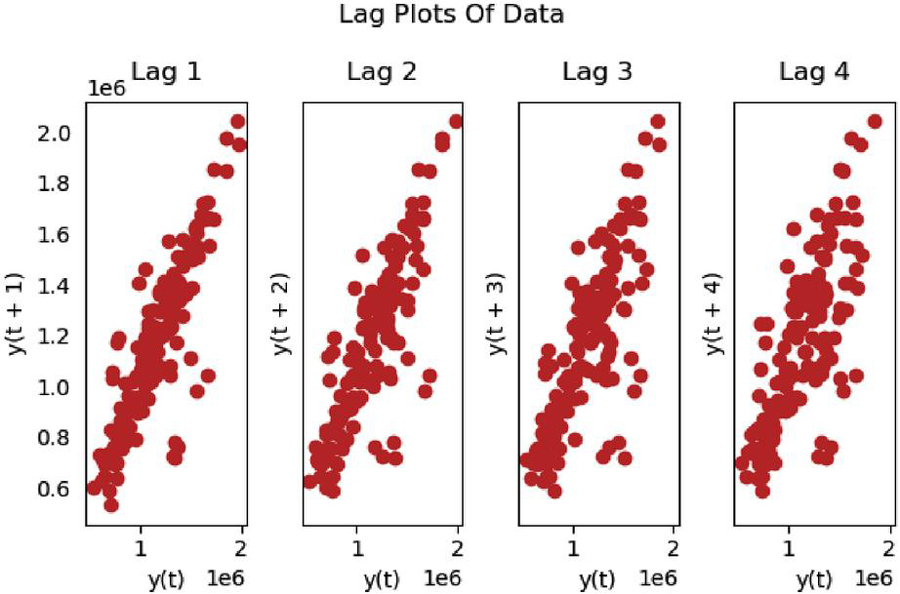Figure 3 Results of lag analysis of the overall electricity consumption of various industries in Yunnan Province.

As can be seen in Figure 3, the data show an overall aggregation trend as the lag value increases, indicating that the data we used have a strong autocorrelation.

#### 3.2.2 Lag analysis

We examined the data for trend and seasonal characteristics in order to further study the characteristics of the experimental data, and the results are displayed in Figure 4.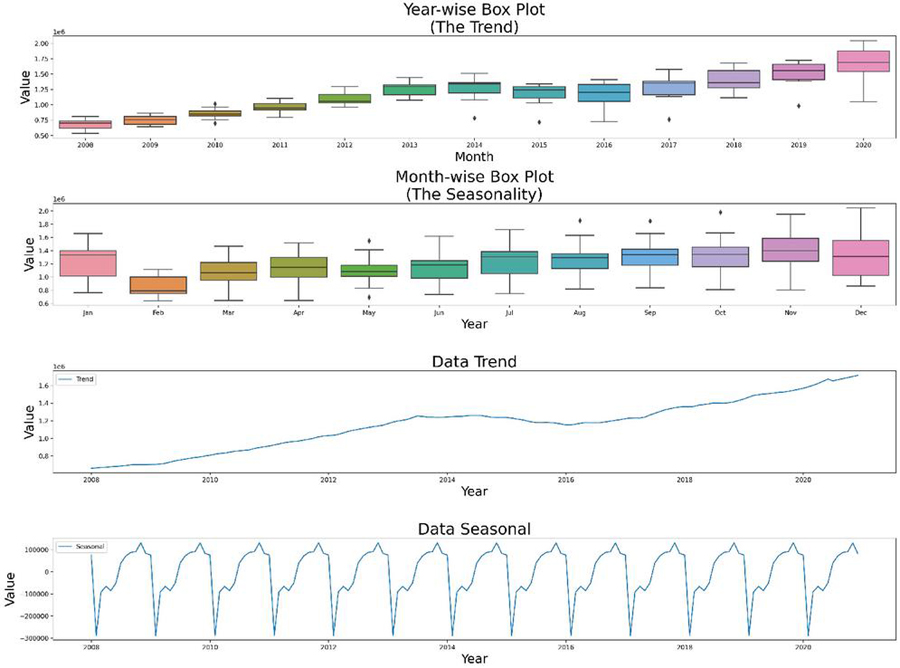Figure 4 Results of trend and seasonal characteristics analysis of electricity consumption of the overall electricity consumption of various industries in Yunnan Province.

It can be seen clearly from Figure 4 that the experimental data have a strong trend and show a continuous growth trend, the experimental data have strong seasonality, and the seasonal characteristics of the experimental data are considered to be extracted with an emphasis in the subsequent study.

#### 3.2.3 Data stability test

The ADF test (Augmented Dickey-Fuller Testing) was applied to the experimental data to determine whether they have predictive value. The visualization outcomes after computing the rolling mean and rolling standard deviation are displayed in Figure 5.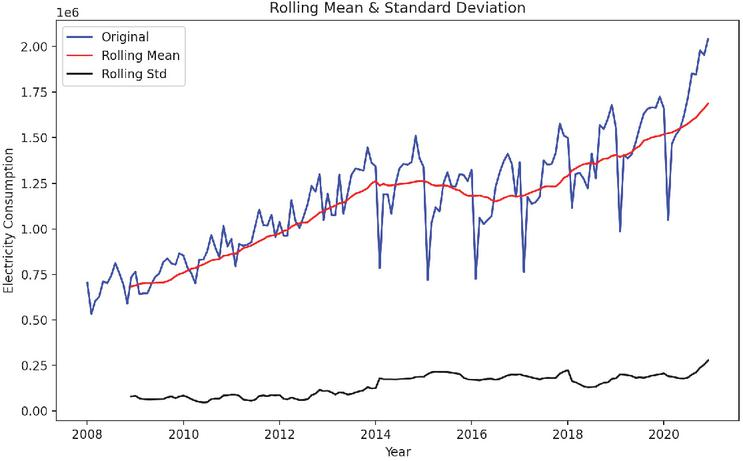Figure 5 Results of smoothness test of electricity consumption of the overall electricity consumption of various industries in Yunnan Province.Figure 6 Difference conversion of electricity consumption of the overall electricity consumption of various industries in Yunnan Province.

It can be seen in Figure 5 that the rolling mean is a trend component, although the rolling standard deviation is relatively constant over time. To ensure the time series is smooth, we need to ensure that the rolling statistics remain constant over time, so their curves must all be parallel to the x-axis. Next, we perform ADF test. After testing, we found that the time series of the experimental data is not stationary, so we decided to use some stationary time series methods such as logarithmic transformation, exponential transformation and time split to better determine the model parameters. The result of the data differential conversion is shown in Figure 6.

These techniques considerably smoothed out the time series, and we used the ADF test to measure the impact of data modification. The results of the ADF test are displayed in Table 1.

Table 1 Results of ADF test for difference conversion of electricity consumption of the overall electricity consumption of various industries in Yunnan Province

 Indicator Value Test Statistic 4.722616 p-value 7.6e-5 #Lags Used 12.0 Number of Observations Used 142.0 Critical Value (1%) 3.477262 Critical Value (5%) 2.882118 Critical Value (10%) 2.577743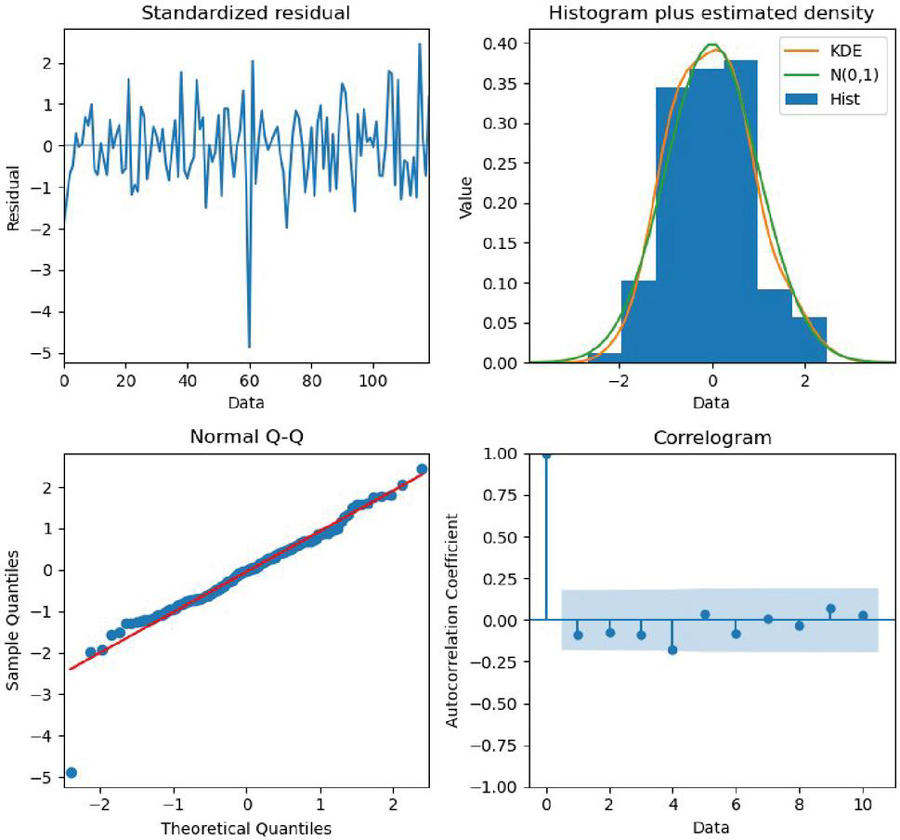Figure 7 Parameter optimization of SARIMA-based M-LTLF model.

As can be seen above, the p-value is reduced from 0.99 to 0.000076. Other values are also closer to the test statistic. This proves that the time series has been extremely smooth and the next step of optimizing the parameters can be carried out.

#### 3.2.4 Optimal parameter selection

We make the time series smooth by first-order difference, so the time difference order d is set to 1 and the period difference order D is set to 1. The time series has a very strong seasonal character by year, so the periodic time interval S is set to 12.

From the ACF and PACF plots, we can determine the values of the optimal parameters. We can consider temporarily taking p to be 1 and q to be 1. After that, we use the optimal parameter selection method to determine the maximum lag order p of the non-seasonal average operator to be 0, the maximum lag order q of the autoregressive average operator to be 1, the maximum lag order P of the seasonal average operator to be 0, and the maximum lag order Q of the moving average operator to be 2.

### 3.3 Analysis of Forecast Results

We use the electricity consumption of the overall electricity consumption of various industries in Yunnan Province from 2008 to 2018 for model training, and use the electricity consumption of the overall electricity consumption of various industries in Yunnan Province from 2019 to 2020 for testing the model performance. The final model fitting results are displayed in Figure 8.Figure 8 Results of SARIMA-based M-LTLF.

To test the forecast results, an accuracy assessment was performed, and the MAPE was selected as the evaluation criterion of the model in this paper. The model MAPE error was calculated to be 6.05%.

To further test the effectiveness of the forecast, we test the forecast model.

(1) Firstly, the standardized residual plots were drawn to check whether the SARIMA-based M-LTLF model could make forecasts correctly, specifically by checking whether the residuals of the model conformed to the characteristics of the normal distribution through the standardized residual plots, as shown in Figure 9.Figure 9 Standardized residual plots of SARIMA-based M-LTLF model.

The histogram of the model residual distribution and the model kernel density curve obtained after running the model are shown in Figure 10, which clearly shows that the standardized residuals of the forecast model can show a normal distribution.Figure 10 Histogram of residual distribution and kernel density curve of SARIMA-based M-LTLF model.

(2) Then, we looked into the possibility that the model’s residuals might exhibit correlation properties. In particular, we produced the Q-Q distribution of the forecast model residuals and the ACF of the model residuals (shown in Figure 11). The ACF plot demonstrates that the autocorrelation function and partial autocorrelation function of the residual series of the model are essentially within the acceptable error range. The Q-Q distribution demonstrates that the distribution of the residuals follows the trend of normal distribution and has the characteristics of normal distribution.Figure 11 Q-Q distribution and residual ACF of SARIMA-based M-LTLF model.

### 3.4 Comparative Analysis

We have used more than ten models in the experimental process and selected three of them with better results for cross-sectional comparison. The MAPE of SARIMA, HOLT_WINTER, LSTM, and ARIMA models are 6.05%, 9.18%, 10.67%, and 13.87%, respectively, which shows that the model we proposed is significantly more effective than the others.

MAPE is a relative error measure that is suitable for performance comparisons of different time series forecasting models, so we use MAPE as a metric for the model optimizer.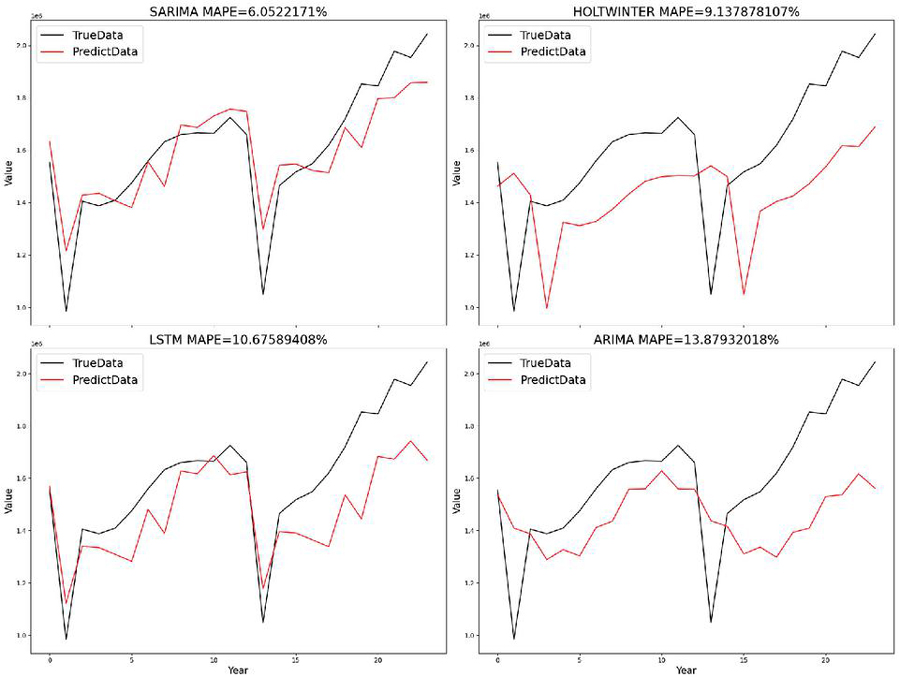Figure 12 Experimental results of comparing electricity consumption data of the whole society in Yunnan Province.

## 4 Conclusion

In our study, a SARIMA model for M-LTLF is constructed by modeling the electricity consumption data of the whole society in Yunnan Province from 2008 to 2018. Firstly, the experimental data were pre-processed and analyzed by using lag analysis, trend and seasonal characteristics analysis, and data stability test, and the analysis results show that the experimental data have the characteristics of strong autocorrelation, continuous upward trend, and strong seasonality; then, log transformation, exponential transformation, and time splitting were used to make the experimental data smooth; finally, the parameters of the model were optimized, and the model was tested using the test set, and the model finally obtained a MAPE of 6.05%.

By comparing the forecast results with the models of ARIMA, HOLT_WINTER and LSTM, we find that the model we proposed outperforms the other models compared in the paper and creates a new idea for M-LTLF.

## Acknowledgments

The research is supported by the Special Plan of Yunnan Province Major Science and Technology Plan, China(202102AA100021), the Demonstration Project of Comprehensive Government Management and Large-Scale Industrial Application of The Major Special Project of CHEOS (89-Y50G31-9001-22/23), the Yunnan Natural Science Foundation, China(202101AT070167), a grant from Key Laboratory for Crop Production and Smart Agriculture of Yunnan Province.

## Funding

 Number Acronym Sponsor 1 SPYPMSTP The Special Plan of Yunnan Province Major Science and Technology Plan, China No.202102AA100021. 2 DPCGMLSIAAMSPCHEOS The Demonstration Project of Comprehensive Government Management and Large-Scale Industrial Application of The Major Special Project of CHEOS No.289-Y50G31-9001-22/23. 3 YNSFC The Yunnan Natural Science Foundation, China No.202101AT070167. 4 KLCPSAYP The Key Laboratory for Crop Production and Smart Agriculture of Yunnan Province.

## References

 Zheng Lei, Shuxin Tian, Dawei Yan, Lianbin Wei, Kui Wang and Lu Liu. (2020). Mid-Long Term Load Forecasting Based on ARIMA-TARCH-BP Neural Network Model. Chinese Journal of Electron Devices, 43(01), 175–179.

 Pinjing Zou, Jiangang Yao, Weihui Kong, Linbo Hu and Xueqing Pan. (2017). Mid-long Term Power Load Forecasting Based on Multivariable Time Series Inversion Self-memory Model. Proceedings of the CSU-EPSA, 29(10), 98–105.

 Xincheng Sun, Jianshou Kong and Zhao Liu. (2018). Middle-term power load forecasting model based on kernel principal component analysis and improved neural network. Journal of Nanjing University of Science and Technology, 2018, 42(03), 259–265.

 Zheng Zheng, Lei Tan, Nan Zhou, Junwei Han, Jing Gao and Liguo Weng. Power load prediction based on multi-headed attentional convolutional network. Journal of Nanjing University of Information Science and Technology(Natural Science Edition).

 Yongzhi Wang, Bo Liu and Yu Li. (2020). A Power Load Data Prediction Method Based on LSTM Neural Network Model. Research and Exploration in Laboratory, 39(05), 41–45.

 Zhaoying Tu, Yi Mao, Xiaoxiao Song and Junliang Yuan. (2019). Research on Medium and Long Term Power Load Forecasting in Boluo County. Guangdong Province, 42(01), 76–81.

 Hui Hou, Qing Wang, Bo Zhao, Leiqi Zhang, Xixiu Wu and Changjun Xie. (2022). Power load forecasting without key information based on phase space reconstruction and machine learning. Power System Protection and Control, 50(04), 75–82.

 Zuleta-Elles, I., Bautista-Lopez, A., Cataño-Valderrama, M. J., Marín, L. G., Jiménez-Estévez, G., and Mendoza-Araya, P. (2021, December). Load Forecasting for Different Prediction Horizons using ANN and ARIMA models. In 2021 IEEE CHILEAN Conference on Electrical, Electronics Engineering, Information and Communication Technologies (CHILECON) (pp. 1–7). IEEE.

 Yang, Z. C. (2016). Discrete cosine transform-based predictive model extended in the least-squares sense for hourly load forecasting. IET Generation, Transmission and Distribution, 10(15), 3930–3939.

 Saxena, H., Aponte, O., and McConky, K. T. (2019). A hybrid machine learning model for forecasting a billing period’s peak electric load days. International Journal of Forecasting, 35(4), 1288–1303.

 Maldonado, S., Gonzalez, A., and Crone, S. (2019). Automatic time series analysis for electric load forecasting via support vector regression. Applied Soft Computing, 83, 105616.

 Liu, Z., Sun, X., Wang, S., Pan, M., Zhang, Y., and Ji, Z. (2019). Midterm power load forecasting model based on kernel principal component analysis and back propagation neural network with particle swarm optimization. Big data, 7(2), 130–138.

 Gao, W., Darvishan, A., Toghani, M., Mohammadi, M., Abedinia, O., and Ghadimi, N. (2019). Different states of multi-block based forecast engine for price and load prediction. International Journal of Electrical Power and Energy Systems, 104, 423–435.

 Yaoyao He, Yang Qin and Shanlin Yang. (2019). Medium-term power load probability density forecasting method based on LASSO quantile regression. Systems Engineering-Theory and Practice, 39(07), 1845–1854.

 Jiangyong Liu, Wenhan Liu and Lingzhi Yi. (2020). Multi-sequence Coordinated Medium-term Load Forecasting Model. Proceedings of the CSU-EPSA, 32(02), 48–53.

 Jun Liu, Hongyan Zhao, Jiacheng Liu, Liangjun Pan and Kai Wang. (2019). Medium-term Load Forecasting Based on Cointegration-Granger Causality Test and Seasonal Decomposition. Automation of Electric Power Systems, 43(01), 73–80.

 Shan Jiang, Qiupeng Zhou, Hongchuan Dong, Xu Ma and Zhenyu Zhao. Long-term load combination forecasting method considering the periodicity and trend of data. Electrical Measurement and Instrumentation.

 Box, G. E., and Pierce, D. A. (1970). Distribution of residual autocorrelations in autoregressive-integrated moving average time series models. Journal of the American statistical Association, 65(332), 1509–1526.

 Jingli Guo and Bo Dong. (2019). International rice price forecast based on SARIMA model. Price:Theory and Practice, (01), 79–82.

 Yanqun Sun, Shougang Zhang, Moyuan Lu, Yan Zhang, Yanyu Pan, Chong Wang, Qixin Wu, Meixue Yao and Chengguo Li. (2022). Prediction of mosquito infestation in Nanjing based on SARIMA model. Journal of Nanjing Medical University(Natural Sciences), 42(01), 108–111.

 Xingqiang Pan, Rui Ma, Tianchi Yang, Yi Chen, Keqin Ding and Guozhang Xu. (2022). Establishing a seasonal ARIMA prediction model of varicella incidence in Ningbo city using Python programming language. Chinese Journal of Vaccines and Immunization, 28(01), 83–87$+$104.

 Guoyun Zhang and Hui Jin. (2022). Research on the prediction of short-term passenger flow of urban rail transit based on improved ARIMA model. Computer Applications and Software, 39(01), 339–344.

## BiographiesChunlin Yin received his M. S. degree from Yun Nan University in 2017. He is currently work for the Electric Power Research Institute of Yunnan Power Grid as an engineer. His main research interests are transfer learning.Kaihua Liu is currently a bachelor’s student at Yunnan University. His main research fields are Machine Learning and Computer Vision.Qiangjian Zhang received his B.S. degree in industrial engineering from Kunming University in 2019. He is currently a master’s student of Yunnan University. His main research fields are Machine Learning and Computer Vision.Kai Hu is currently work for Yunnan Power Grid Co., Ltd. as an engineer. His main research interests are Power System Analysis and Planning.Zheng Yang received his M. S. degree from Beijing Jiao Tong University in 2012. He is currently work for the Electric Power Research Institute of Yunnan Power Grid as an engineer. His main research interests are computer networking and security.Li Yang received her MA.Eng degree from Kunming University of Science and Technology in 2011. She is currently work for the Electric Power Research Institute of Yunnan Power Grid as an senior engineer. Her main research interests are digital transformation.Na Zhao received the Ph.D. degree from the Yunnan University in 2011. She is an Associate Professor with the School of Software, Yunnan University. Her research interests include software engineering, complex network, the Internet of things, smart grid, etc.